# Complex Powers of Complex Numbers

Having looked at issues surrounding powers and roots of complex numbers, including fractional powers, let’s go even further and consider complex powers of complex bases. Things will get a little weird as we work toward $$(2+3i)^{3+2i}$$!

## Powers and logs of a negative  base

We’ll start with a basic question from 1997 about real powers, which will open the door to complex numbers:

Exponentiation

How do I calculate x^y using only exp, ln, log, and the trigonometric functions?  I know exp(y*ln(x)) works, but only for x > 0.

We could calculate, say, $$2^3$$, as $$\left(e^{\ln(2)}\right)^3=e^{3\ln(2)}=e^{3\cdot0.693…}=e^{2.079…}=8.$$ But to do this for $$(-2)^3$$, we’d have to take the log of a negative number, which isn’t defined. Yet we know the answer, $$-8$$.

Doctor Rob answered, pointing out how we can still use the natural log, but it will be imaginary and non-unique:

The key is DeMoivre's Law:

e^(i*t) = cos(t) + i*sin(t),

where i is the square root of -1, that is, i^2 = -1.  Even if x and y are real, ln(x) may not be, because x may be negative.  What is ln(x)? It is a number z such that x = exp(z).  In fact, there may be several values of z that work.  From DeMoivre's Law, we see that

-1 = cos(Pi) + i*sin(Pi) = e^(i*Pi),

so ln(-1) = i*Pi.  This is not the only answer, however!  It is true that

-1 = cos(Pi + 2*Pi*k) + i*sin(Pi + 2*Pi*k) = e^(i*Pi*[2*k+1]),

so ln(-1) = i*Pi*(2*k+1), for any integer k.  This should remind you of inverse trigonometric functions, which also have multiple values. Using this fact, and the definition you started with, x^y = e^(y*ln(x)), and the properties of logarithms, you should be able to define x^y for all real x and y now.

We saw this deMoivre’s theorem in Complex Numbers: Multiplication and Rotation. Here, we’re turning it inside-out, finding the inverse of the exponential function. Here are some of the (infinitely many!) natural logs of -1, which are odd multiples of $$\pi i$$: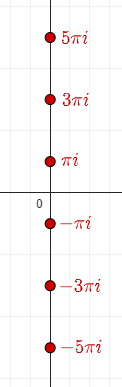When we raise e to any of these values, we rotate 1 by an angle coterminal with 180°, to -1. The same happens for the natural log of any number, once you allow values off the real line.

Let's try

(-2)^(1/2) = e^((1/2)*ln(-2))
= e^([ln(-1) + ln(2)]/2)
= e^([i*Pi + 2*Pi*i*k + ln(2)]/2)
= e^(i*Pi/2 + Pi*i*k + ln(2)/2)
= [cos(Pi/2 + Pi*k) + i*sin(Pi/2 + Pi*k)]*2^(1/2)
= [0 + i*(-1)^k]*2^(1/2)
= (-1)^k*i*Sqrt
= + or - i*Sqrt

This seems to make sense.  You even get both signs!

That is, $$\left(-2\right)^{1/2}=e^{\ln(-2)/2}=\\e^{\frac{\ln(-1)+\ln(2)}{2}}=\\e^{\frac{i\pi+2\pi i\cdot k+\ln(2)}{2}}=\\e^{\left(\frac{\pi}{2}+\pi k\right)i}e^{\frac{\ln(2)}{2}}=\\\left(\cos\left(\frac{\pi}{2}+\pi k\right)+i\sin\left(\frac{\pi}{2}+\pi k\right)\right)e^\frac{\ln(2)}{2}=\\\left(0+i\left(-1\right)^ k\right)\left(2\right)^\frac{1}{2}=\\\left(-1\right)^ k i\sqrt{2}=\pm i\sqrt{2}$$ which is just what we expected.

Notice how the infinite number of logs of $$-2$$, shown below, collapse into just two powers of -1, resulting in the two square roots.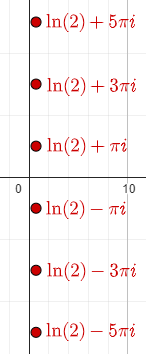Furthermore, this same method can be used to define x^y when both are complex numbers, although it may be a multi-valued "function."

i^i = e^(i*ln(i)),
= e^[i*(Pi*i/2 + 2*Pi*i*k)], for any integer k
(because  e^(Pi*i/2) = cos(Pi/2) + i*sin(Pi/2) = i),
= e^[-Pi/2 - 2*Pi*k], for any integer k.

It seems paradoxical that i^i should be real, but that is correct.

Again, writing this to be easier to read, we have $$i^i=e^{i\ln(i)}=\\e^{i\left(\frac{\pi}{2}i+2\pi i k\right)}=\\e^{-\frac{\pi}{2}-2\pi k}$$ Since $$e^{-\pi/2}\approx0.208$$ and $$e^{2\pi}\approx535.5$$, these are spaced too widely to graph them reasonably!

Let’s back up a bit and let only the exponent be imaginary, before jumping back in with both feet:

## Any real number to the i th power

We got this question in 2000:

Complex Numbers to Complex Powers

I understand Euler's discovery of e^ix and its relation to the sine and cosine functions. But since e is just a number (although a special one), what is the general value of n^i? Specifically, since a real number to a real power equals a real, does a real number to a complex power equal some complex number? If so, what is it?

Hi, Michael.

You're right; in fact, we can use the formula to raise any complex number to any complex power.

Any real number a can be written as e^ln(a); so

a^(ix) = (e^ln(a))^(ix)
= e^(ix*ln(a))
= cos(x*ln(a)) + i*sin(x*ln(a))

We can extend this to complex exponents this way:

a^(x+iy) = a^x * a^(iy)

(I should have excluded $$a=0$$. But $$a=1$$ turns out to be interesting.)

To give specific examples, $$2^i=\cos(\ln(2)+i\sin(\ln(2))\approx0.769+0.639i$$ and $$2^{3+4i}=2^3\left(\cos(4\ln(2)+i\sin(4\ln(2))\right)\approx-7.4615+2.8855i$$

To allow for complex bases, write the base in the form a*e^(ib), and you find

[a*e^(ib)]^z = a^z * e^(ib*z)

These ideas will allow you to raise any real or complex base to any real or complex exponent.

We’ll do this later.

### Wrapping the plane around the origin

Michael responded:

Doctor Peterson,

I asked what the result was of raising a real by a complex number. You sent me a great reply, which is understandable on an analytical level. However, when viewed geometrically, it seems that result of this operation maps onto a unit circle on the complex plane.

This seems too fantastic. I've relied on intuition for my sense of mathematics, but this is beyond my senses. How is it possible that all combinations of real numbers raised to powers with only an imaginary component degenerate to the unit circle?

If I had to make up an explanation I might say that in the world of numbers, reals cause translation and imaginaries cause rotation; also, since multiplying by i is often seen as rotating by 90 degrees in the complex plane, then by extension, raising by an imaginary is just a more complicated version of this rotation characteristic of i. Is this possible?

Thank you.

What he says here is said in the post I mentioned above, about multiplication and rotation. (But multiplication by reals  produces not translation, which represents addition, but stretching, or dilation.)

I replied:

Yes, you're right: any real raised to any pure imaginary power gives a complex number with absolute value 1, so the mapping y -> e^(iy) takes the real line onto the unit circle, wrapping it around with a period of 2 pi. That's what Euler's formula means: raising e to an imaginary power produces the complex number with that angle.

Here is that “wrapped number line” ($$e^{-y}$$) corresponding to imaginary exponents, in red, and the ray ($$e^{x}$$) corresponding to real exponents, in green: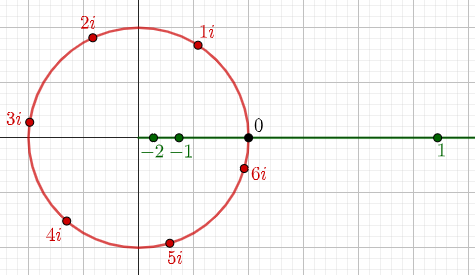And the entire complex plane maps onto the plane under the transformation $$f(z)=e^{z}$$.

These lines representing values of z,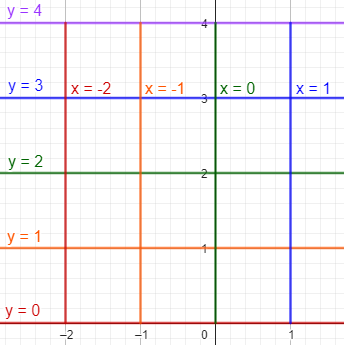are mapped to these values of $$f(z)=e^{z}$$: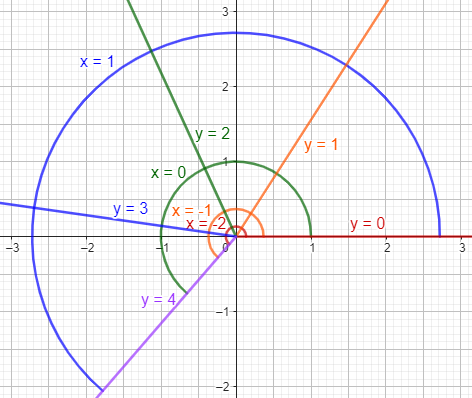The green arc is the image of the imaginary axis, and the red ray is the image of the entire real axis.

For example, the value $$z=1+2i$$, represented by point $$(1,2)$$, is mapped to $$f(1+2i)=e^{1+2i}=e^{1}\left(\cos(2)+i\sin(2)\right)\approx-1.13+2.47i.$$

You may want to look at our FAQ on this,

Imaginary Exponents and Euler's Equation
http://mathforum.org/dr.math/faq/faq.euler.equation.html

When we raise a real to a complex power, the imaginary component of the exponent rotates it while the real component dilates (not translates) it. That is, the angle of the resulting number is determined by the imaginary part of the exponent, while the absolute value is determined by the real part. This is what gives Euler's formula tremendous power, and in fact this is the answer to the often-asked question, what good are complex numbers? They let us combine dilation and rotation, or exponential and sinusoidal functions, into a single operation.

This is discussed in Euler’s Formula: Complex Numbers as Exponents.

Multiplication by a complex number, similarly, rotates by the angle of the complex number (adding the two angles), and dilates by its absolute value (multiplying the absolute values.)

So now let’s do that!

## Complex number to complex powers, all the way

Now we’ll jump in the deep end, with a question from Jacob in 2015:

Complex Exponentiation

Okay, I have a "biggie" for you.

I am trying (and failing) to raise a complex number to a complex power.

Actually, the different cases involving complex powers with and without base-e have been scattered across your archives, so I was thinking I'd make it easier for the next person to find an answer all in one place. (Also, I'm not really sure I'm doing half of them right.) Would you mind explaining all four cases?

I know Euler's Identity, (e^(xi) = cos(x) + isin(x)), and the natural logarithm identity a = e^(ln(a)). But I have been looking for MANY hours on how to do this (mainly the last one), and I cannot find a decent explanation of how to do it by hand (well, not COMPLETELY by hand...).

But mostly, I'm having trouble solving x^y when BOTH x and y are complex numbers.

Okay, here are my four example cases.

I DO think that I got this one right:

e^(3i)
= cos(3) + i sin(3)
= -.989992 + .141120i

I think that I got this one right, too:

e^(2 + 3i)
= e^2*e^(3i)
= e^2*(cos(3) + i sin(3))
= -7.315110 + 1.042743i

I'm not sure if I got this one right:

6^(2 + 3i)
= e^(ln(6^(2 + 3i)))
= e^((2 + 3i)ln(6))
= e^(2ln(6))*e^(3iln(6))
= 36*(cos(3ln(6)) + i sin(3ln(6)))
= 22.154288 - 28.375825i

Here's the one that I really can't seem to figure out:

(2 + 3i)^(3 + 2i)
= e^(ln((2 + 3i)^(3 + 2i)))
= e^((3 + 2i)ln(2 + 3i))
= e^((3 + 2i)^(ln(2 + 3i))) ...

And that's where I can't continue.

I know you can't split it into its respective parts, because you're raising the WHOLE base (in this case, 3 + 2i) to the power (in this one, ln(2 + 3i)).

The most difficult part about these, I think, is the fact that you're breaking apart everything you've learned. I guess we have Euler to thank for making it even possible; but multiplying a number by itself a non-real number of times already strains the intuition.

Can you help me out here? For the life of me, I cannot understand this one. I'd appreciate it forever.

I'm one of those people who can't sleep until they figure out the problem that they've started.

Any help at all is appreciated! Thank you!!

(I’ve corrected errors that weren’t mentioned in the replies.)

Doctor Ali answered first, with a link to the previous question, but Jacob asked for more, because we hadn’t actually done the work for that general case. He gave it a start:

Hi Jacob!

Thanks for writing to Dr. Math.

Let's say that we want to find z1^z2, where

z1 = a + b i
z2 = c + d i

This is the most general case in raising complex numbers to complex powers. We can write it as

(a + bi)^(c + di) = (a + bi)^c * (a + bi)^(di)

The first part, (a + bi)^c, is pretty easy. If c is an integer, you'll just need to use the binomial expansion and expand (a + bi)^c and do the calculation, bearing in mind that i^2 = -1. If it's not an integer, you'll need to write (a + bi) in polar form and use De Moivre's Formula to find the answer in polar form. That is,

a + bi = r exp(i t)

So,

(a + bi)^c = (r exp(i t))^c = r^c exp(i t c)

The binomial expansion would not be easy even for larger integer exponents; we’ll mostly be using de Moivre.

Now for the second part, we want to evaluate (a + bi)^(di). We can write it as

(a + bi)^(di) = ((a + bi)^d)^i

The term (a + bi)^d is just like above. Now, you have a complex number that you want to raise to i-th power. You can easily do this using the same method discussed in the below page from our archive:

http://mathforum.org/library/drmath/view/53860.html

I will let you do this part yourself as an exercise. What you are supposed to do is to find M and N in the expression below:

(x + yi)^i = M + N i

Once you master this technique, you'll see that you can directly separate the real part and imaginary part of the power in the original question and use De Moivre's Formula to find the result of each.

Please write back if you still have any difficulties.

The link is to a 1999 answer on raising real numbers to complex powers (particularly, the ith power and ith root), using the same method we’ve seen.

### Getting through the details

Jacob still struggled:

Hi Dr. Ali,

I apologize for the late reply. I've had a lot going on.

I did have a chance to check out the links, and they explain a lot to me. However, I still am not sure how to get past a certain part in solving

(2 + 3i)^(3 + 2i)

I can get this far:

(-46 + 9i)(-5 + 12i)^i

But from there, I have no idea where to go. I didn't see that they did it in those other answered questions, either. I know you can't "distribute" the exponent (in this case, i), and I don't think you can make a logarithm base -5 + 12i of (x/(-46 - 9i)).

And I obviously can't write out and multiply -5 + 12i an i number of times....

This is driving me crazy!

Thanks for all of your help!

Jacob

PS. I saw in another article that you can take the i index of a number (basically, raise it to a (1/i) power). I don't really understand how they got the answers that they did. Can you explain that to me (or send me a link with the work written out)?

I'm so sorry, I've created more questions for you.

Thank you so much!!

His work would look like this: $$(2+3i)^{3+2i}=(2+3i)^3(2+3i)^{2i}=\\\left(2^3+3(2^2)(3i)+3(2)(3i)^2+(3i)^3\right)\left((2+3i)^2\right)^i=\$$8+36i-54-27i)\left(2^2+2(2)(3i)+(3i)^2\right)^i=\\(-46+9i)\left(4+12i-9\right)^i=\\(-46+9i)\left(-5+12i\right)^i So the work was well done as far as it goes; I’ll finish this work at the end, and we’ll see how close he came. I took on the challenge, doing it a different way (using polar form for the base from the start, but rectangular form for the exponent): Hi, Jacob. Here is what I would do to find (2 + 3i)^(3 + 2i). We first have to express 2 + 3i in polar form. We find that the angle (argument) is arctan(3/2), which I will just call A for now to simplify my work, and the length (modulus) is sqrt(2^2 + 3^2) = sqrt(13). Thus, 2 + 3i = sqrt(13))*e^(Ai) = e^(ln(sqrt(13)) + Ai)  When we have a complicated constant expression, it is a nice idea to give it a name so you don’t have to write it too often; here I chose A for angle (above), and R for Real part (below): Let's use R to represent ln(sqrt(13)), so 2 + 3i = e^(R + Ai) Now, (2 + 3i)^(3 + 2i) = (e^(R + Ai))^(3 + 2i) = e^((R + Ai)(3 + 2i)) = e^((3R - 2A) + (2R + 3A)i) = e^(3R - 2A)*e^((2R + 3A)i) = e^(3R - 2A)[cos(2R + 3A) + sin(2R + 3A)i] = e^(3R - 2A)cos(2R + 3A) + e^(3R - 2A)sin(2R + 3A)i  Here is the work in more readable form: (2+3i)^{3+2i}=\left(e^{R+Ai}\right)^{3+2i}=e^{(R+Ai)(3+2i)}=\\e^{(3R-2A)+(2R+3A)i}=e^{3R-2A}\cdot e^{(2R+3A)i}=\\e^{3R-2A}[\cos(2R+3A)+\sin(2R+3A)i]=\\e^{3R-2A}cos(2R+3A)+e^{3R-2A}sin(2R+3A)i Numerically, A = arctan(3/2) = 0.98279 R = ln(sqrt(13)) = 1.28247 3R - 2A = 1.88183 e^(3R - 2A) = 6.5655 2R + 3A = 5.51333 cos(2R + 3A) = 0.718012 sin(2R + 3A) = -0.6960310 So our result is 6.5655*0.718012 + 6.5655*-0.6960310i = 4.714 - 4.569i Does that give you a better sense of where an answer like this comes from? Today, we can just ask Wolfram Alpha to evaluate (2 + 3i)^(3 + 2i) for us: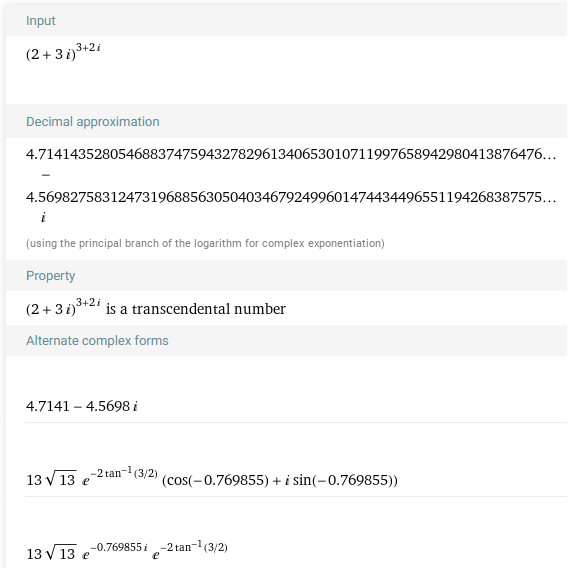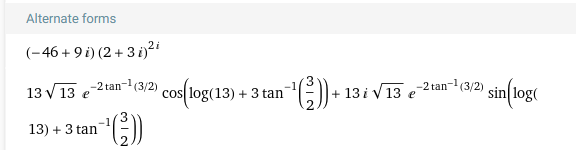Jacob replied, Again, sorry for the late response. I get it now. Thank you so much! This is awesome!! I really appreciate all that you have done for me. It means so much. I wish you the best! Happy Math! Jacob ### Finishing Jacob’s work Jacob had stopped at (-46+9i)\left(-5+12i\right)^i. Let’s see if we can do the last step in what Jacob did, raising a complex number to the ith power. The base, \(-5+12i$$, has angle $$A=\pi-\arctan\left(\frac{12}{5}\right)$$ (because it is in the second quadrant), and modulus $$\sqrt{(-5)^2+12^2}=13$$. So$$\left(-5+12i\right)^i=\left(13e^{Ai}\right)^i=13^i\left(e^{Ai}\right)^i=13^ie^{-A}
=\\e^{-A}e^{\ln\left(13\right)i}=e^{-\pi+\arctan\left(12/5\right)}\left(\cos\left(\ln13\right)+i\sin\left(\ln13\right)\right)\approx\\-0.1401(-0.8383+0.5452i)=0.1174-0.0764i(-46+9i)\left(-5+12i\right)^i\approx(-46+9i)(-0.1174+0.0764i)=4.71-4.57i

And we got the same result.

This site uses Akismet to reduce spam. Learn how your comment data is processed.